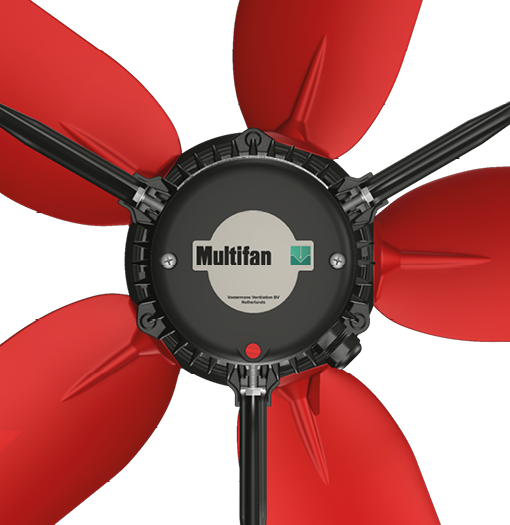# The fan laws

2 minutes reading - published on December 10, 2019The operation of a fan is subject to some mechanical laws. What happens if the speed changes due to regulating with a frequency controller?

• If the speed is changed, the air volume will change proportionally.
• The pressure (∆ PStat) that the fan can handle, corresponding to the air volume, will increase or decrease quadratically with the speed.
• The power consumption will change to 3rd power if the speed changes.
##### An example

Assume: rpm = 1,400 rpm, volume = 10000 m³/h with a ∆ Pstat of 80 Pa and a power consumption Pin = 500 watts.

Now the speed drops by 50%, so to 700 rpm. Then the volume = 5,000 m³/h with a ∆ Pstat of 20 Pa and a power consumption Pin = 62.5 watts. The new values for each point on the fan curve can now be calculated. This creates a valid curve for the new speed.

##### What can we do with this?

If we do not use the maximum recommended pressure of a fan, but adjust it to the desired pressure in the system, then you can save a considerable amount of energy.

The disadvantage is that the yield also decreases and therefore more fans are needed. In the example at the beginning of this article we saw that if we need 20 Pa we use only 1/8 of the power at ½ of the air volume. So, in order to get 100% air again, we need 2 fans that run at 50% and together use 25% of the previously absorbed power!

##### What happens if the weight of the air to be transported changes?

The weight of the air (ρ) can also change, for example, in areas where it is warmer or colder or in places with a different geographical height. If ρ changes (larger or smaller than the standard weight ρ = 1.2 kg/m³), the power consumption will change proportionally to the new ρ. The same applies to the pressure (∆ PStat). This changes proportionally with the new ρ, while the volume remains the same.

Assume: ρ = 1.2 kg/m³

Air volume = 10,000 m³/h with an ∆ Pstat of 80Pa and Pin = 500 watts

Now we are at a different height or we have a different temperature so that ρ = 0.6 kg/m³.

Air volume remains 10,000 m³/h with an ∆ Pstat of 40Pa and Pin = 250 watts

The resistance line has the same properties when ρ changes. The pressure changes equally with the new ρ with a constant air volume.

Related topic: The fan characteristics (Or Q / h curve)

#### Share with otherspublished on December 10, 2019

## We are happy to work with you